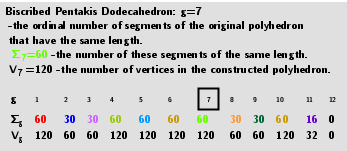# The Great Rhombicosidodecahedron (V=120) from Biscribed Pentakis Dodecahedron for the case of trisection of its 7th-order segments

A polyhedron is constructed whose V=120 vertices are the points of the trisection of the segments the same length 7th-order(g=7) of the Biscribed Pentakis Dodecahedron. Geometric Constructions are in Applet: Series of polyhedra obtained by trisection (truncation) different segments of the original polyhedron, and the resulting polyhedra in Applet: Serie of polyhedra obtained by trisection (truncation) segments of the Biscribed Pentakis Dodecahedron.## 3. Properties of polyhedra

 Vertices: 120  (120) Faces: 62  (30 squares + 20 regular hexagons + 12 regular decagons) Edges: 180
Disdyakis Triacontahedron: Vertices: 62 (30 + 20 + 12) Faces: 120 (acute triangles) Edges: 180 (60 short + 60 medium + 60 long)